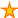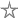# The differences between Nothing, Empty, Missing, Null, Zero and ZLS

22 votes:15,420 views      No comments
by Allen Browne, 18 May 2009    (for Access 97+)

### Tips for Serious Users

Provided by Allen Browne,  March 2009

# Nothing? Empty? Missing? Null?

It can be confusing to know which of these terms to use. They all mean different things, and are not the same as 0 (zero) or "" (a zero-length string.)

Other than Null, they are all part of the VBA language (Visual Basic for Applications.) Null is a term used in all databases; it is the value of a field when you have not entered. Think of it as meaning 'unknown.' Another article deals with the common errors with Null.

The text below will make most sense if you try the examples (right) as you go.

## Nothing

 Nothing is the uninitialized state of an object variable. An object cannot be a simple variable such as a number or a string, so it can never be 0 or "". It must be a more comprehensive structure (a text box, form, recordset, querydef, ...) Since it is not a simple value, you cannot test if it is equal to something. VBA has an Is keyword that you use like this: ```Function TestObject() Dim obj As Object If obj Is Nothing Then Debug.Print "Yep: an object starts out as nothing." End If End Function``` (You get an error if you use = in place of Is for objects.) You can de-assign an object by setting it back to Nothing: `Set Printer = Nothing` Nothing: an uninitialized object

## Empty

 A Variant can act as any type of data: number, string, object, array, user-defined, and so on. You can assign it a simple value: ```Dim var1 As Variant var1 = 0 var1 = ""``` You can assign it an object: ```Set var1 = Forms!Form1 Set var1 = CurrentDb()``` You can assign it an array of values: `var1 = Array(1,2,4)` When first declared, VBA initializes a Variant to a value that behaves as both a 0 (zero) and a "" (a zero-length string): ```Dim var1 As Variant If var1 = 0 Then Debug.Print "The uninitialized variant behaves as zero." End If If var1 = "" Then Debug.Print "The uninitialized variant behaves as a ZLS." End If``` The value that is equal to both a zero and a zero-length string is called Empty. If you try this in the Immediate Window (Ctrl+G), both lines return True: ```? Empty = 0 ? Empty = ""``` That's what Empty means. Note that you cannot normally compare 0 to "", as they are different data types: `? 0 = "" 'Error 13: type mismatch.` The variant is not initialized to behave as an object: ```Dim var1 As Variant If var1 Is Nothing Then 'Error 424: object required.``` But it could be Nothing if you explicitly Set it to an object type, e.g.: ```Set var1 = Nothing If var1 Is Nothing Then MsgBox "It is now.``` Empty: an uninitialized variant

## Missing

 You can write VBA functions that accept optional arguments, like this one where you must supply 2 values, and can supply a third: ```Function DoIt(a, b, Optional c) Debug.Print a Debug.Print b Debug.Print c End Function ``` In the Immediate Window (Ctrl+G), try: `? DoIt(1, "hello")` A prints as numeric value 1.B prints as the string, "hello".C prints as Error 448 (which means 'Named argument not found.') ` ` Since we passed in only 2 arguments the 3rd one is Missing. So, Missing is actually an error value, and you will get an error if you try to do anything with it. VBA provides the IsMissing() function so you can avoid the error by testing for it like this: `If Not IsMissing(c) Then Debug.Print c` Since Missing is an error value, this gives the same result: `If Not IsError(c) Then Debug.Print c` Note that *only* a Variant can be Missing. In the example above, we did not declare any data type for the 3 arguments (a, b, and c), so VBA treats them as variants. If we had declared c as any other VBA type, it would not be Missing, but would be the initial value for that type. This example will yield 'A = 0' when you supply no argument, because the VBA initializes the integer to zero, so it is not Missing: ```Function DoIt2(Optional a As Integer) If IsMissing(a) Then Debug.Print "A is missing" 'never happens Else Debug.Print "A = " & a End If End Function``` Missing: an omitted argument (variant)

## Summary

• An uninitialized Variant is Empty (a value that behaves as both 0 and "".)
• Only the Variant can be Missing (when an argument is omitted.) It has no use except to test if it IsMissing(), since any other use results in an error.
• Nothing is the state of an object that has not been assigned to anything. You can set a Variant equal to an object, so it can be Nothing.
• Only the Variant can be Null (other types will error.) A Null does not equal anything: neither 0, nor "", nor Empty, nor another Null.

 Home Index of tips Top

Rate this article:This is a cached tutorial, reproduced with permission.